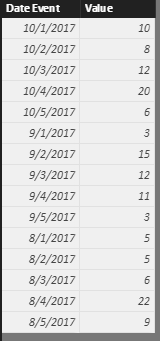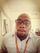cancel
Showing results for
Did you mean:Frequent Visitor

## How to get a value of the same day from previous month?

Hello guys,

I have a problem that I wish to solve, if anyone could help. I tried to calculate a value of the same day, from previous month and I don't have a clue about how to do it. The comparation that I wish to show is the sales of 05-sep-2017 subtracting 05-aug-2017, in a line chart opened by day, for example. It wuold be something like this:

test = Calculate(SUM([Value]) ,  DATEADD([Date Event].[Day]))

Calculate(SUM([Value]) ,  DATEADD([Date Event].[Day], -1, month))

Can anybody help with a solution?

1 ACCEPTED SOLUTIONMicrosoft

Hi @dcrios,

I created a sample table based on my assumption.```Last Month =
CALCULATE (
SUM ( [Value] ),
FILTER (
ALL ( 'Month-Sales' ),
MONTH ( 'Month-Sales'[Date Event] )
= MONTH ( MAX ( 'Month-Sales'[Date Event] ) ) - 1
&& DAY ( 'Month-Sales'[Date Event] ) = DAY ( MAX ( 'Month-Sales'[Date Event] ) )
)
)

difference = MAX('Month-Sales'[Value])-[Last Month]```Best regards,
Yuliana Gu

Community Support Team _ Yuliana Gu
If this post helps, then please consider Accept it as the solution to help the other members find it more quickly.
5 REPLIES 5Microsoft

Hi @dcrios,

I created a sample table based on my assumption.```Last Month =
CALCULATE (
SUM ( [Value] ),
FILTER (
ALL ( 'Month-Sales' ),
MONTH ( 'Month-Sales'[Date Event] )
= MONTH ( MAX ( 'Month-Sales'[Date Event] ) ) - 1
&& DAY ( 'Month-Sales'[Date Event] ) = DAY ( MAX ( 'Month-Sales'[Date Event] ) )
)
)

difference = MAX('Month-Sales'[Value])-[Last Month]```Best regards,
Yuliana Gu

Community Support Team _ Yuliana Gu
If this post helps, then please consider Accept it as the solution to help the other members find it more quickly.Frequent Visitor

Great work, thanx it solves my pblm too, can u please explain in step by stepSuper User

Hi,

Drag the date column to the visual filter and apply a criteria of October 5.  Then write this calculated field formula

`=CALCULATE(SUM([Value]),PREVIOUSMONTH(Calendar[Date]))`

You should have a calendar table with running dates.  There should be a relationship from the Date column of your source data table to the Date column of your calendar table.

Regards,
Ashish Mathur
http://www.ashishmathur.comFrequent Visitor

@Ashish_Mathur my calendar table has future dates as we have set targets till 2019. This seems not to work .Super User

Hi,

Please describe your question in detail.  Share some data and show the expected result.

Regards,
Ashish Mathur
http://www.ashishmathur.com